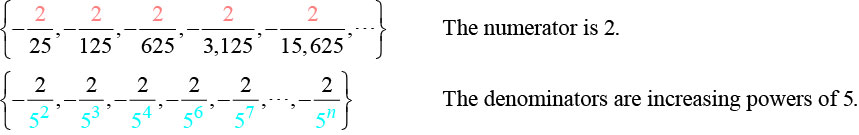# 13.1 Sequences and their notations  (Page 3/15)

 Page 3 / 15

## Writing the terms of an alternating sequence defined by an explicit formula

Write the first five terms of the sequence.

${a}_{n}=\frac{{\left(-1\right)}^{n}{n}^{2}}{n+1}$

Substitute $n=1,$ $n=2,$ and so on in the formula.

$\begin{array}{lll}n=1\hfill & \begin{array}{cc}& \end{array}\hfill & {a}_{1}=\frac{{\left(-1\right)}^{1}{2}^{2}}{1+1}=-\frac{1}{2}\hfill \\ n=2\hfill & \begin{array}{cc}& \end{array}\hfill & {a}_{2}=\frac{{\left(-1\right)}^{2}{2}^{2}}{2+1}=\frac{4}{3}\hfill \\ n=3\hfill & \begin{array}{cc}& \end{array}\hfill & {a}_{3}=\frac{{\left(-1\right)}^{3}{3}^{2}}{3+1}=-\frac{9}{4}\hfill \\ n=4\hfill & \begin{array}{cc}& \end{array}\hfill & {a}_{4}=\frac{{\left(-1\right)}^{4}{4}^{2}}{4+1}=\frac{16}{5}\hfill \\ n=5\hfill & \hfill & {a}_{5}=\frac{{\left(-1\right)}^{5}{5}^{2}}{5+1}=-\frac{25}{6}\hfill \end{array}$

The first five terms are $\left\{-\frac{1}{2},\frac{4}{3},-\frac{9}{4},\frac{16}{5},-\frac{25}{6}\right\}.$

In [link] , does the (–1) to the power of $n$ account for the oscillations of signs?

Yes, the power might be $n,n+1,n-1,\text{\hspace{0.17em}}$ and so on, but any odd powers will result in a negative term, and any even power will result in a positive term.

Write the first five terms of the sequence:

${a}_{n}=\frac{4n}{{\left(-2\right)}^{n}}$

The first five terms are

## Investigating piecewise explicit formulas

We’ve learned that sequences are functions whose domain is over the positive integers. This is true for other types of functions, including some piecewise functions . Recall that a piecewise function is a function defined by multiple subsections. A different formula might represent each individual subsection.

Given an explicit formula for a piecewise function, write the first $\text{\hspace{0.17em}}n\text{\hspace{0.17em}}$ terms of a sequence

1. Identify the formula to which $n=1$ applies.
2. To find the first term, $\text{\hspace{0.17em}}{a}_{1},\text{\hspace{0.17em}}$ use $\text{\hspace{0.17em}}n=1\text{\hspace{0.17em}}$ in the appropriate formula.
3. Identify the formula to which $\text{\hspace{0.17em}}n=2\text{\hspace{0.17em}}$ applies.
4. To find the second term, $\text{\hspace{0.17em}}{a}_{2},\text{\hspace{0.17em}}$ use $\text{\hspace{0.17em}}n=2\text{\hspace{0.17em}}$ in the appropriate formula.
5. Continue in the same manner until you have identified all $\text{\hspace{0.17em}}n\text{\hspace{0.17em}}$ terms.

## Writing the terms of a sequence defined by a piecewise explicit formula

Write the first six terms of the sequence.

Substitute $\text{\hspace{0.17em}}n=1,n=2,\text{\hspace{0.17em}}$ and so on in the appropriate formula. Use ${n}^{2}$ when $n$ is not a multiple of 3. Use $\frac{n}{3}$ when $n$ is a multiple of 3.

The first six terms are

Write the first six terms of the sequence.

The first six terms are

## Finding an explicit formula

Thus far, we have been given the explicit formula and asked to find a number of terms of the sequence. Sometimes, the explicit formula for the $\text{\hspace{0.17em}}n\text{th}\text{\hspace{0.17em}}$ term of a sequence is not given. Instead, we are given several terms from the sequence. When this happens, we can work in reverse to find an explicit formula from the first few terms of a sequence. The key to finding an explicit formula is to look for a pattern in the terms. Keep in mind that the pattern may involve alternating terms, formulas for numerators, formulas for denominators, exponents, or bases.

Given the first few terms of a sequence, find an explicit formula for the sequence.

1. Look for a pattern among the terms.
2. If the terms are fractions, look for a separate pattern among the numerators and denominators.
3. Look for a pattern among the signs of the terms.
4. Write a formula for ${a}_{n}$ in terms of $n.$ Test your formula for and $n=3.$

## Writing an explicit formula for the n Th term of a sequence

Write an explicit formula for the $n\text{th}$ term of each sequence.

1. $\left\{-\frac{2}{11},\frac{3}{13},-\frac{4}{15},\frac{5}{17},-\frac{6}{19},\dots \right\}$
2. $\left\{-\frac{2}{25}\text{,}-\frac{2}{125}\text{,}-\frac{2}{625}\text{,}-\frac{2}{3\text{,}125}\text{,}-\frac{2}{15\text{,}625}\text{,}\dots \right\}$
3. $\left\{{e}^{4}\text{,}{e}^{5}\text{,}{e}^{6}\text{,}{e}^{7}\text{,}{e}^{8}\text{,}\dots \right\}$

Look for the pattern in each sequence.

1. The terms alternate between positive and negative. We can use $\text{\hspace{0.17em}}{\left(-1\right)}^{n}\text{\hspace{0.17em}}$ to make the terms alternate. The numerator can be represented by $n+1.$ The denominator can be represented by $2n+9.$

${a}_{n}=\frac{{\left(-1\right)}^{n}\left(n+1\right)}{2n+9}$

2. The terms are all negative.So we know that the fraction is negative, the numerator is 2, and the denominator can be represented by ${5}^{n+1}.$

${a}_{n}=-\frac{2}{{5}^{n+1}}$
3. The terms are powers of $e.$ For $n=1,$ the first term is ${e}^{4}$ so the exponent must be $n+3.$

${a}_{n}={e}^{n+3}$

#### Questions & Answers

write down the polynomial function with root 1/3,2,-3 with solution
if A and B are subspaces of V prove that (A+B)/B=A/(A-B)
write down the value of each of the following in surd form a)cos(-65°) b)sin(-180°)c)tan(225°)d)tan(135°)
Prove that (sinA/1-cosA - 1-cosA/sinA) (cosA/1-sinA - 1-sinA/cosA) = 4
what is the answer to dividing negative index
In a triangle ABC prove that. (b+c)cosA+(c+a)cosB+(a+b)cisC=a+b+c.
give me the waec 2019 questions
the polar co-ordinate of the point (-1, -1)
prove the identites sin x ( 1+ tan x )+ cos x ( 1+ cot x )= sec x + cosec x
tanh`(x-iy) =A+iB, find A and B
B=Ai-itan(hx-hiy)
Rukmini
what is the addition of 101011 with 101010
If those numbers are binary, it's 1010101. If they are base 10, it's 202021.
Jack
extra power 4 minus 5 x cube + 7 x square minus 5 x + 1 equal to zero
the gradient function of a curve is 2x+4 and the curve passes through point (1,4) find the equation of the curve
1+cos²A/cos²A=2cosec²A-1
test for convergence the series 1+x/2+2!/9x3ByByByBy Mistry BhaveshBy